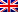#[HackingWeek 2015] [Crypto3] Write-up

## Description

Alice wants to use the ECDSA signature algorithm.
For this, she chooses to use the elliptic curve equation E where y2 = x3 – x, defined on the finite field with q elements, Fq, with q = 134747661567386867366256408824228742802669457 .

She chose P as a base point of order N = 2902021510595963727029 with:
P = (18185174461194872234733581786593019886770620, 74952280828346465277451545812645059041440154)

Then, she chooses as secret signature key a random integer d with 1<d<N.
The public key is the point Q=d⊗P where ⊗ is the scalar multiplication on the elliptic curve E.

The value of Q is:
Q=(76468233972358960368422190121977870066985660, 33884872380845276447083435959215308764231090)

The validation key is given by the value of d.